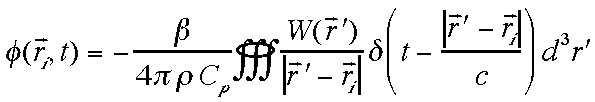# Velocity Potential related to energy deposition

Now consider time-resolved velocity potential at a position distant from the site of energy depostion. The following equation is based on the thermoelastic wave equation:where δ() indicates a Dirac delta function whose value is zero unless t = (|r' - ri|/cs), and cs is speed of sound. The subscript i refers to the ith detector in an array of detectors. The term d3r' refers to an incremental volume element dV in the volume integration.

W(r',t) directly contributes to φ(ri,t), attenuated by a 1/r dependence, 1/(|r' - ri|). The summation of contributions (W)(dV)/(|r' - ri|) from all positions whose contribution would arrive at r at time t yields the velocity potential at position r and time t. Such an integration can gather the contributions from various objects or from various parts of an extended irregular object. The φ(ri,t) is calculated as a function of time by repeated application of the above integral at different time points t. Finally, the time derivative of φ(ri,t) times -ρ yields the time-resolved pressure P(r,t).

Contributions to pressure P may be either positive or negative due to compressive and tensile components. The interference of positive and negative pressure waves arriving at a point of observation can be a difficult task to compute. However, by doing the bookkeeping in terms of the velocity potential, the problem is simple integration with a subsequent time derivative which is relatively simple to implement.